Function Repository Resource:

# EquationOfStateConstants

Calculate the constants associated with an equation of state

Contributed by: Jan Mangaldan
 ResourceFunction["EquationOfStateConstants"]["name","eos"] gives the values of the constants associated with the equation of state "eos" for the chemical "name". ResourceFunction["EquationOfStateConstants"][entity,"eos"] gives the values of the constants for the given entity. ResourceFunction["EquationOfStateConstants"][assoc,"eos"] uses the association assoc to look up properties needed to compute the constants.

## Details

An equation of state is a thermodynamic equation that relates state variables (e.g. pressure, volume and temperature) and describes the state of matter under a given set of physical conditions.
The following equations of state are supported:
 "Berthelot" Berthelot equation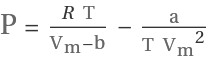"Dieterici" Dieterici equation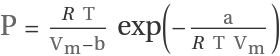"CarnahanStarling" Carnahan-Starling equation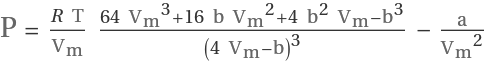"RedlichKwong" Redlich-Kwong equation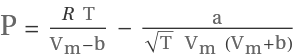"VanDerWaals" van der Waals equation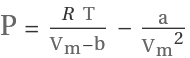In ResourceFunction["EquationOfStateConstants"][assoc,"eos"], assoc must be given as an association containing the following elements:
 "CriticalPressure" critical pressure "CriticalTemperature" critical temperature

## Examples

### Basic Examples (5)

Get the van der Waals constants for argon:

 In:=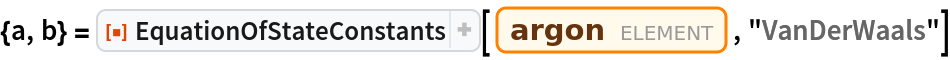Out=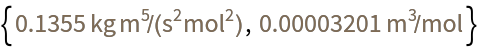Compare with the result of ChemicalData:

 In:=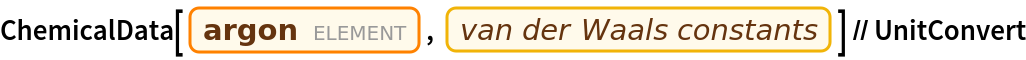Out=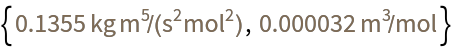Use the van der Waals constants to compute the pressure of argon, given a molar volume of 1 L/mol at a temperature of 1800 °C:

 In:=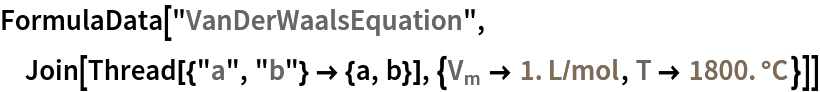Out=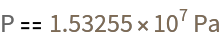Compare with the result of using the ideal gas equation:

 In:=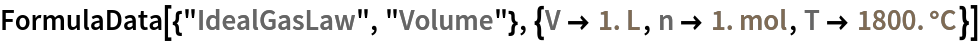Out=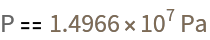Compare with the result of using ThermodynamicData:

 In:=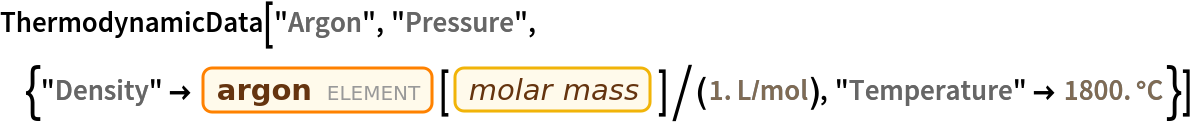Out=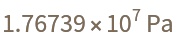### Scope (2)

 In:=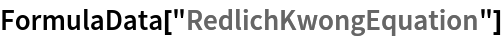Out=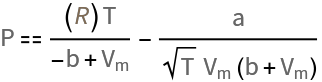Use the Redlich–Kwong equation to compute the molar volume of ethane at standard temperature and pressure:

 In:=Out=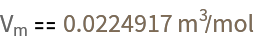Compare with the result of using the van der Waals equation:

 In:=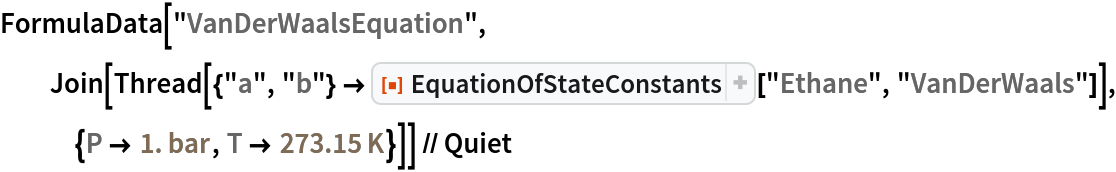Out=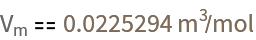Compute the constants for the Dieterici equation for Freon C-318 by supplying explicit values for the critical temperature and pressure:

 In:=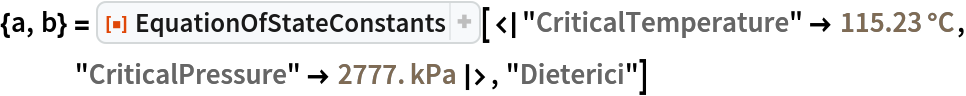Out=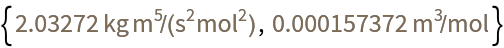Use the constants to compute the pressure at -10 °C of C-318 with a molar volume of 500 mL/mol:

 In:=Out=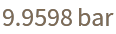### Applications (3)

Compute the Redlich–Kwong constants for a gas mixture that is 70% nitrogen and 30% oxygen by weight, using mixing rules for the constants:

 In:=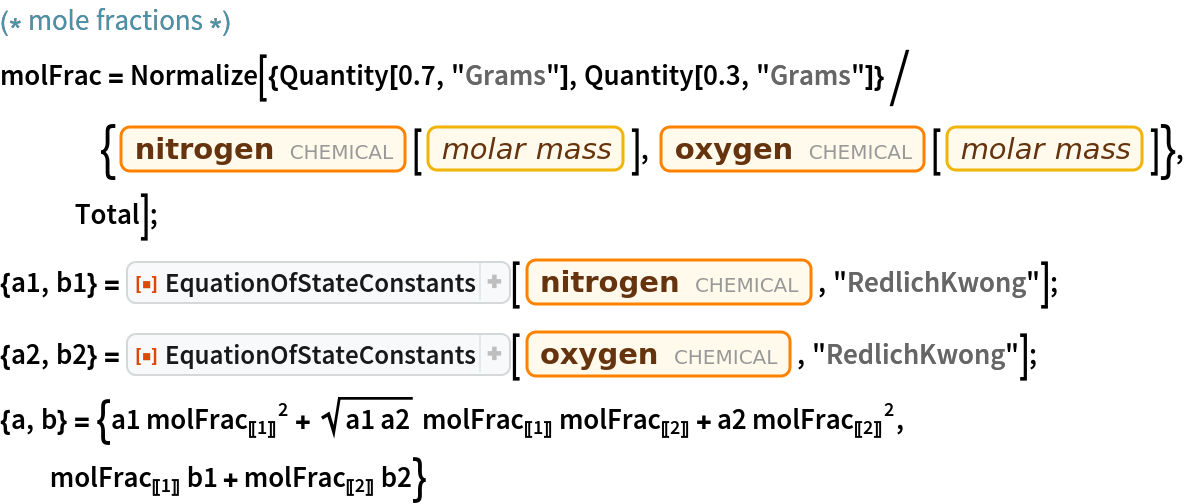Out=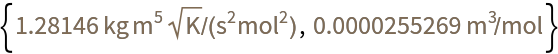Compute the density of the mixture at standard temperature and pressure:

 In:=Out=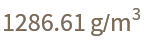Compute the mass of 10 L of the mixture:

 In:=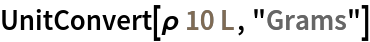Out=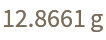## Version History

• 1.0.0 – 14 June 2021